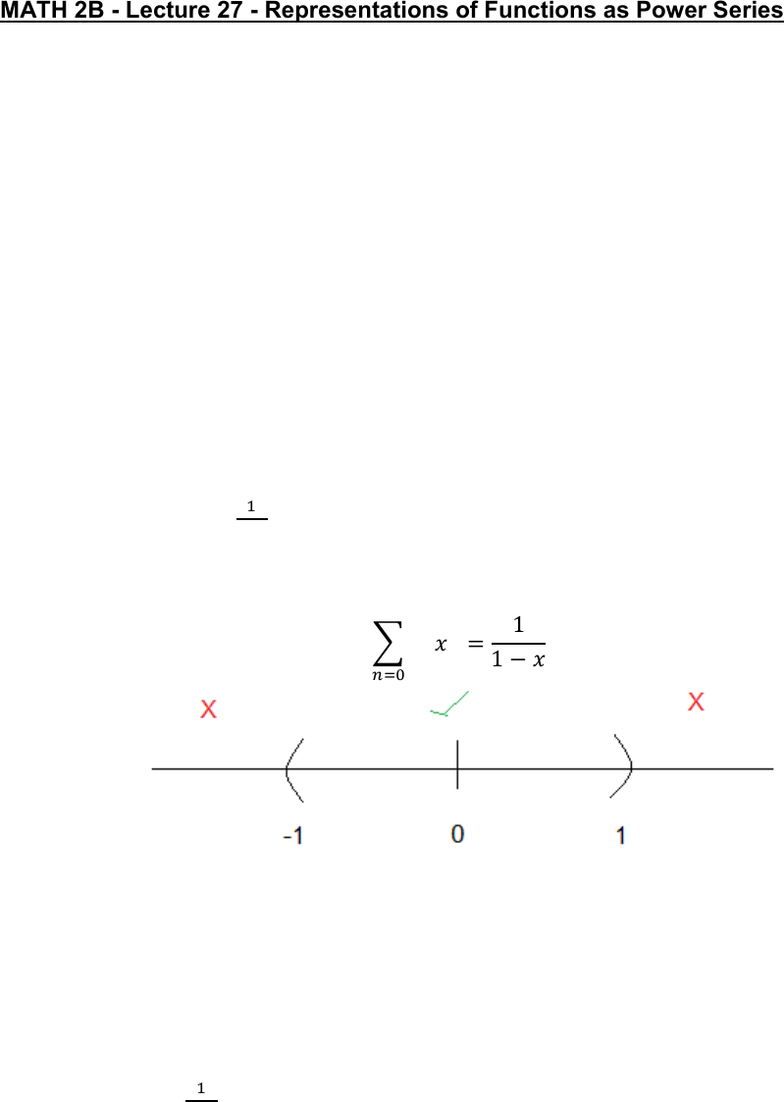Class Notes (1,100,000)
US (460,000)
UC-Irvine (10,000)
MATH (1,000)
MATH 2B (600)
Lecture 27

# MATH 2B Lecture 27: Representations of Functions as Power SeriesPremium

Department
Mathematics
Course Code
MATH 2B
Professor
ERJAEE, G.
Lecture
27

This preview shows half of the first page. to view the full 3 pages of the document.03/08/2019
MATH 2B - Lecture 27 - Representations of Functions as Power Series
We first recall the geometric series
      
Expanding it, we obtain
   

Therefore, we can shift the index n by 1, and obtain a new form for geometric series
     
Now, we consider a simple power series

    
We can treat this power series as a geometric series by letting    
Similarly, we have

      
Therefore, we can think this power series as a function. But this only hold on a
certain interval

So, a power series is equal to a function only on its interval of convergence
Now, we try to represent some functions by using power series, and we also
need to find the interval of convergence
Example:
Represent
 by power series
Solution:
We always need the geometric series